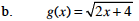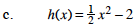### Home > PC > Chapter 1 > Lesson 1.1.3 > Problem1-37

1-37.
1. Find the inverse of each function below. Use correct notation to express your answer. Homework Help ✎

1. f(x) = 3x3 − 5

2. g(x) =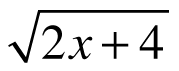3. h(x) =x2 − 2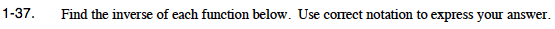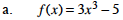Substitute y for the function notation.

Switch every x to y and every y to x.

Solve for y.

Divide both sides by 3.

Take the cube root of both sides:

y = 3x3 − 5

x = 3y3 − 5

$\textit{x} + 5 = 3\textit{y}^{3}$

$\frac{x+5}{3} = \textit{y}^3$

$\small \sqrt\frac{x+5}{3}$